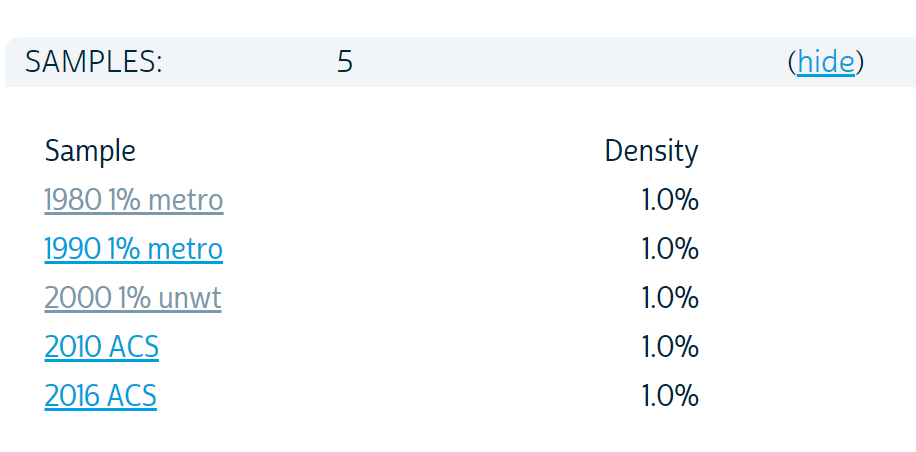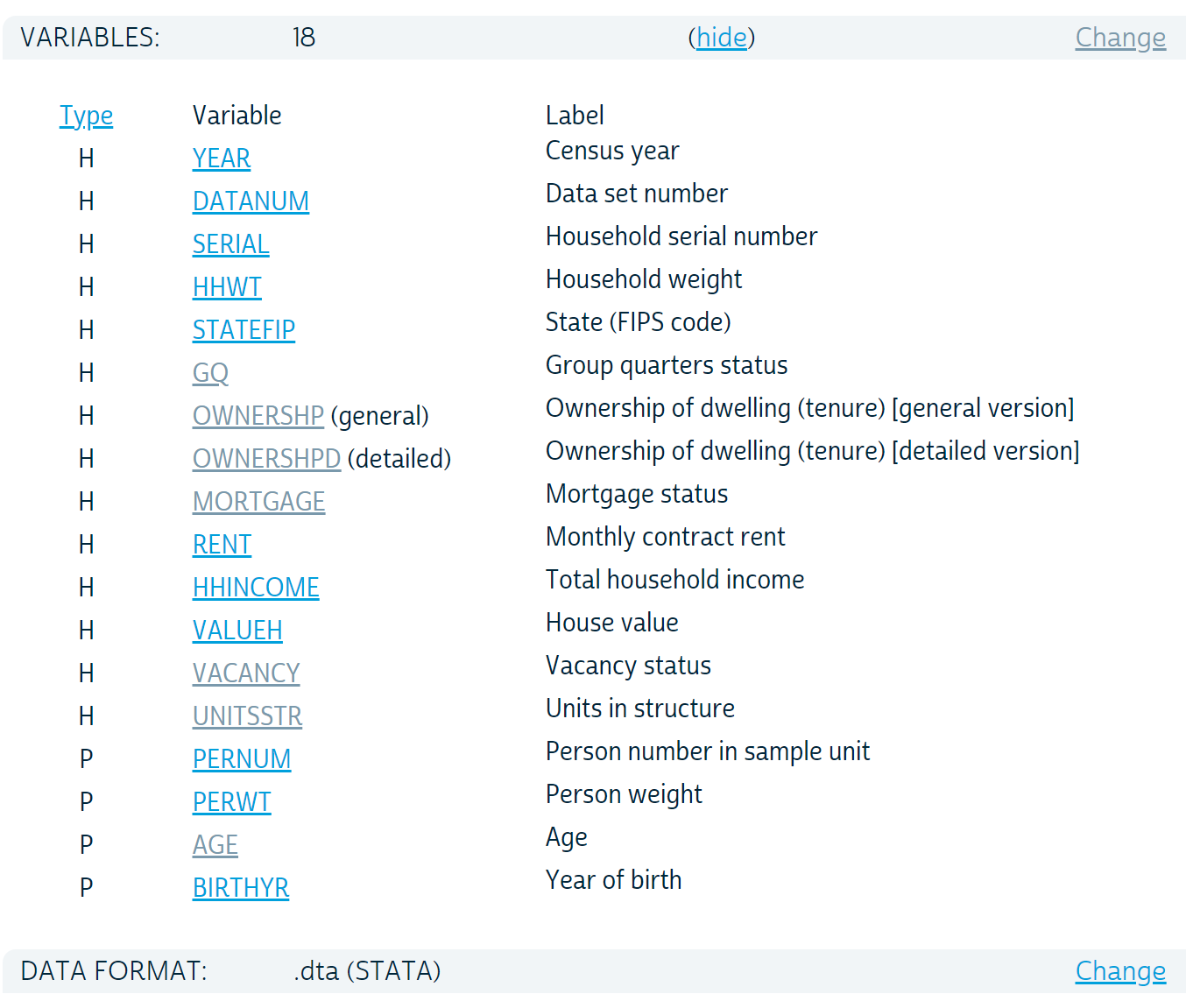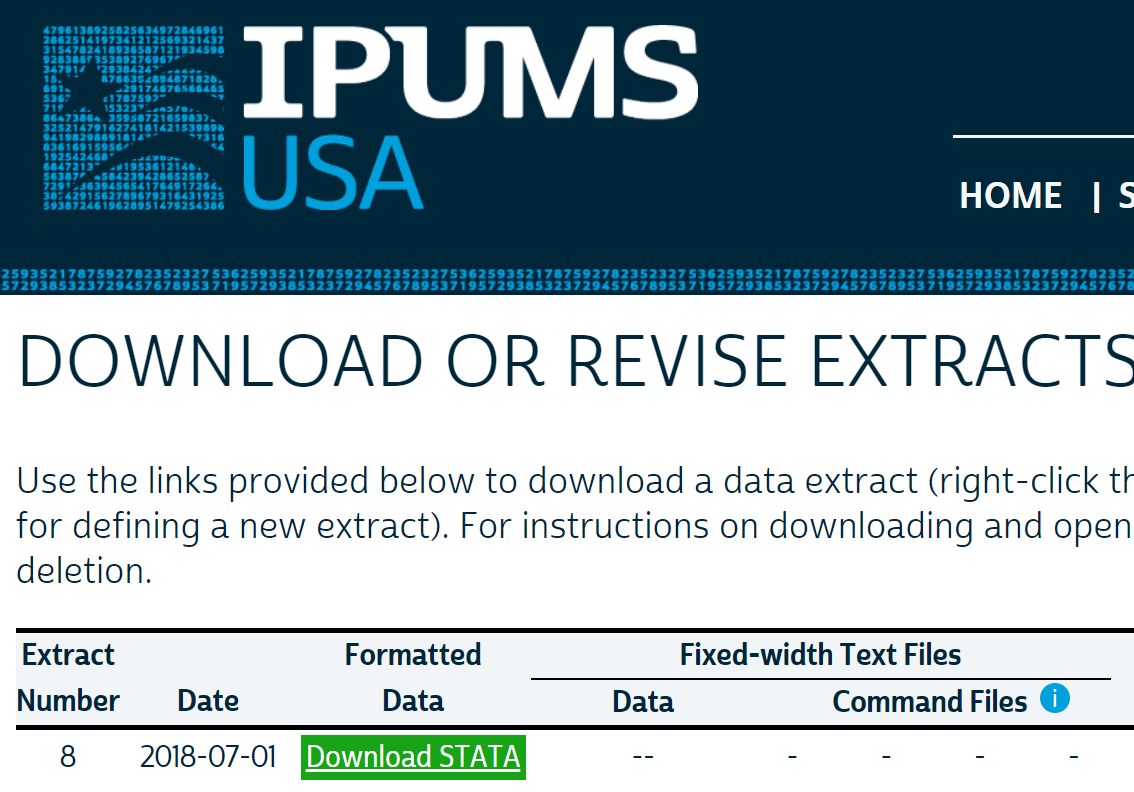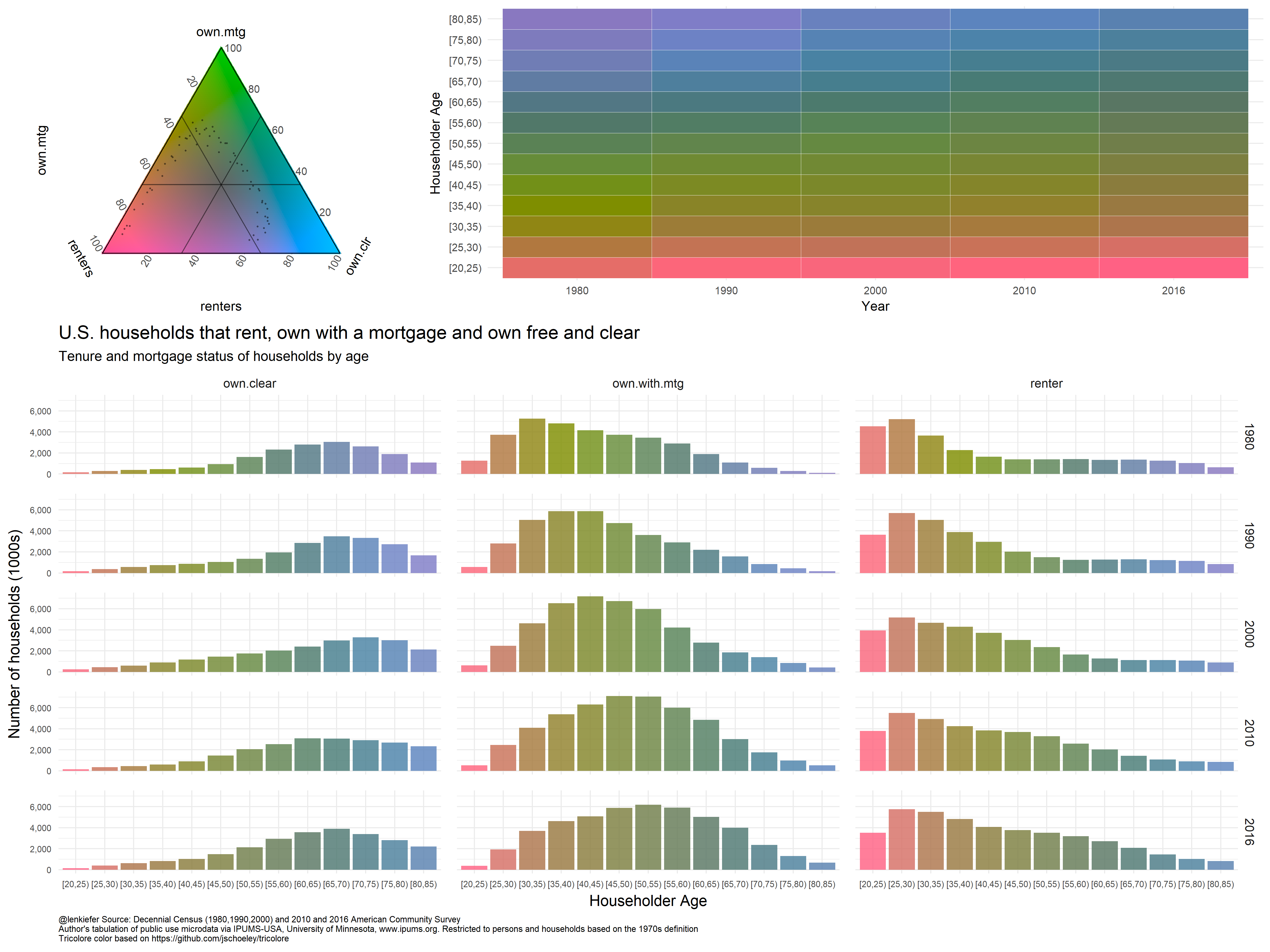# Exploring housing data with R and IPUMS USA

## Explore housing trends in the US, practice some data wrangling and *tri* out a new data visualization technique.

In this post I want to share some observations on housing in the United States from 1980 to 2016, share some R code for data wrangling, and tri (no that’s not a typo, just a pun) out a visualization techniques.

Let’s get to it.

I’ve been carrying a running conversation with folks on Twitter regarding the U.S. housing market and its future. Much of that depends on the evolution of demographic forces. There is a large group of young adults who will drive the U.S. housing market in years to come, but the U.S. also has the aging Baby Boomer generation.

Earlier today I shared this from a presentation by the Federal Reserve Bank of Kansas City’s economist Jordan Rappaport. See here pdf for slides from a recent talk Jordan gave.

The slide I shared shows a U shaped pattern in the share of household who live in apartment based on age. I thought it would be interesting to recreate this chart and then see how this relationship has changed over time. I’ve seen Jordan present a version of this in other presentation, though it’s not in the link above.

# Get data

## IPUMS, you (I)PUMS, we all PUMS for IPUMS

In order to explore these trends, we’re going to need to get some data. As we want custom tabulations, we cannot rely on the ACS package like we did here. Instead we’ll use IPUMS-USA to download data from the U.S. Census Bureau’s Public Use Microdata Samples.

If you want to follow along you’ll need to sign into IPUMS and download your own data. I’ll share the details of the sample I’m using, but the file is too large to post here. I want to see how things may have shifted since 1980, so I’ll collect samples from the 1980, 1990, and 2000 Decennial Census. I’ll also grab the latest available microdata from the 2016 American Community Survey (and 2010 ACS as well). IPUMS has various options you can choose from when selecting your sample, but here are the variables I chose.IPUMS allows you to select only certain variables for analysis. I chose the following variables along with the defaults that include year, group quarters status (GQ) and household (HHWT) and person (PERWT) weights.

• AGE
• Birth Year
• OWNERSHP
• MORTGAGE

I also collected a few other variables that I won’t use here, but we might use at a later date.After you selected your variables you can select the format for your data. They don’t have a R option right now, but that’s okay. We can select a Stata data format and use haven to import into R. Note:, you’ll want to selected a Stata formatted data. Select the data format on the data extract request page. See this FAQ.

You could also use a fixed width format, but I find the Stata format easier to work with thanks to haven. We could also use ipumsr, but I haven’t worked with that package yet.Now that we have our data, we’re ready to rock.

## Wrangle

Normally, when I work with large(ish) data like Census microdata, I would use the data.table package. But for today’s analysis, we’ll restrict ourself to the tidyverse. It turns out for most of what we’re doing the gains from data.table in terms of speed of computation are relatively minor, a few seconds at most for most operations we’ll do here.

Thanks to the fabulous work of IPUMS, our data wrangling is pretty limited. The dataset we got from IPUMS is nice and rectangular. There are a few categorical variables with numeric codes (like ownership, mortgage status and structure type).

First, we’ll load our data into R with haven and then we’ll create three useful variables.

# Load libraries ----
library(tidyverse)
library(haven)
library(data.table)
library(scales)

# create variables
df <- mutate(df,
own  = ifelse(ownershp==1,1,0),
agec = cut(age,seq(0,100,5),right=FALSE),
mtg  = case_when(
mortgage == 0 ~ NA_real_,  # special NA for integers
# https://stackoverflow.com/questions/44893933/avoiding-type-conflicts-with-dplyrcase-when
mortgage == 1 ~ 0,
TRUE ~ 1 )
)

Now, we’ll create two summary datasets. One will be based on households, the other persons. For all analysis we’ll use individuals living outside of group quarters. We’ll use households under the 1970s definition (GQ==1) so we can have consistency from 1980 forward. Though the additional counts you’d get from using the 1990s and 2000s household definitions are relatively minor.

# household level dataframe ----

df.hha <-
df %>%
filter(pernum==1 & gq %in% c(1)) %>%
group_by(year, agec) %>%
summarize(
hh      = sum(hhwt),
renters = sum(ifelse(own==0,hhwt,0)),
owners  = sum(ifelse(own==1,hhwt,0)),
ho=weighted.mean(own,hhwt,na.rm=T),
mtg= weighted.mean(
case_when(
mortgage == 0 ~ NA_real_,
mortgage == 1 ~ 0,
TRUE ~ 1 ),
hhwt, na.rm=T),

agemin = min(age,na.rm=T),
agem = weighted.mean(age, hhwt,na.rm=T),
agemax = max(age,na.rm=T),
byrmin = min(birthyr, na.rm=T),
byrmax = max(birthyr, nar.rm=T),
mf= weighted.mean(ifelse(unitsstr %in% c(7,8,9,10),1,0),hhwt,na.rm=T),
sf=weighted.mean(ifelse(unitsstr %in% c(3,4),1,0),hhwt,na.rm=T)
) %>%
mutate( own.mtg = mtg*owners,
own.clr = (1-mtg)*owners) %>%
arrange(year, agec)

# person level dataframe ----
# won't use this one today, but could be useful if we wanted to do some person-level tabulations

df.pera <-
df %>%
filter( gq %in% c(1)) %>%
group_by(year, agec) %>%
summarize(
pop      = sum(perwt),
renters = sum(ifelse(own==0,perwt,0)),
owners  = sum(ifelse(own==1,perwt,0)),
ho=weighted.mean(own,perwt,na.rm=T),
mtg= weighted.mean(
case_when(
mortgage == 0 ~ NA_real_,
mortgage == 1 ~ 0,
TRUE ~ 1 ),
perwt, na.rm=T),
agemin = min(age,na.rm=T),
agem = weighted.mean(age, perwt,na.rm=T),
agemax = max(age,na.rm=T),
byrmin = min(birthyr, na.rm=T),
byrmax = max(birthyr, nar.rm=T),
mf= weighted.mean(ifelse(unitsstr %in% c(7,8,9,10),1,0),perwt,na.rm=T),
sf=weighted.mean(ifelse(unitsstr %in% c(3,4),1,0),perwt,na.rm=T)
) %>%
mutate( own.mtg = mtg*owners,
own.clr = (1-mtg)*owners) %>%
arrange(year, agec)

That’s it in terms of data wrangling. Let’s make some plots.

### Colors for plots

For most of the plots below, we’ll use a custom scale. We’ll follow this post by Simon Jackson that describes how to create custom color palettes for ggplot2.

  # Function for colors ----
#####################################################################################
## Make Color Scale ----  ##
#####################################################################################
my_colors <- c(
"green"      = rgb(103,180,75, maxColorValue = 256),
"green2"      = rgb(147,198,44, maxColorValue = 256),
"lightblue"  =  rgb(9, 177,240, maxColorValue = 256),
"lightblue2" = rgb(173,216,230, maxColorValue = 256),
'blue'       = "#00aedb",
'red'        = "#d11141",
'orange'     = "#f37735",
'yellow'     = "#ffc425",
'gold'       = "#FFD700",
'light grey' = "#cccccc",
'purple'     = "#551A8B",
'dark grey'  = "#8c8c8c")

my_cols <- function(...) {
cols <- c(...)
if (is.null(cols))
return (my_colors)
my_colors[cols]
}

my_palettes <- list(
main  = my_cols("blue", "green", "yellow"),
cool  = my_cols("blue", "green"),
hot   = my_cols("yellow", "orange", "red"),
mixed = my_cols("lightblue", "green", "yellow", "orange", "red"),
mixed2 = my_cols("lightblue2","lightblue", "green", "green2","yellow","gold", "orange", "red"),
mixed3 = my_cols("lightblue2","lightblue", "green", "yellow","gold", "orange", "red"),
mixed4 = my_cols("lightblue2","lightblue", "green", "green2","yellow","gold", "orange", "red","purple"),
mixed5 = my_cols("lightblue","green", "green2","yellow","gold", "orange", "red","purple","blue"),
mixed6 = my_cols("green", "gold", "orange", "red","purple","blue"),
grey  = my_cols("light grey", "dark grey")
)

my_pal <- function(palette = "main", reverse = FALSE, ...) {
pal <- my_palettes[[palette]]

if (reverse) pal <- rev(pal)

colorRampPalette(pal, ...)
}

scale_color_mycol <- function(palette = "main", discrete = TRUE, reverse = FALSE, ...) {
pal <- my_pal(palette = palette, reverse = reverse)

if (discrete) {
discrete_scale("colour", paste0("my_", palette), palette = pal, ...)
} else {
}
}

scale_fill_mycol <- function(palette = "main", discrete = TRUE, reverse = FALSE, ...) {
pal <- my_pal(palette = palette, reverse = reverse)

if (discrete) {
discrete_scale("fill", paste0("my_", palette), palette = pal, ...)
} else {
}
}

# Tricolore

This is worth exploring in more detail in a future post, but I just learned about the very interesting Tricolore package. This allows you to make charts with a flexible color scale for the visualization of three-part/ternary compositions. It’s like taking bivariate color scales to a whole new dimension.

We happen to have a nice example, with the three types of households we reviewed above (renters, owners with a mortgage, and owners who own free and clear). If we install the package via devtools::install_github('jschoeley/tricolore, we can run the following and get:

# load extra libraries ----
library(ggtern)
library(tricolore)
library(cowplot)

# Might need to run this to avoid breaking ggtern with cowplot
# see https://github.com/wilkelab/cowplot/issues/88
theme_set(theme_gray())

# create complex plot

hha.test <- filter(df.hha,agemin< 85, agemin>19) %>% mutate(r=(1-ho), m=(1-ho)*mtg, f=(1-ho)*(1-mtg))

#df.tri <- Tricolore(hha.test, "r","m","f")

df.tri <- Tricolore(hha.test, "renters","own.mtg","own.clr")
hha.test$srgb <- df.tri$hexsrgb  # merge colors back on data

df.tri <- Tricolore(hha.test, "renters","own.mtg","own.clr")
hha.test$srgb <- df.tri$hexsrgb  # merge colors back on data

g.tri <-
df.tri\$legend +
theme(plot.background = element_rect(fill = NA, color = NA))#+
# labs(x="",y="")

g.tile <-
ggplot(data=hha.test,
aes(x=agec,
y=factor(year),
fill=srgb))+
geom_tile(color="white")+
labs(x="Householder Age", y="Year")+
scale_fill_identity()+
coord_flip()

# put triangle legend with tile plot
g3 <- plot_grid(g.tri,g.tile,rel_widths=c(1,2))

df.bar3 <- left_join(df.bar, select(hha.test, year,agec,srgb), by=c("year","agec"))

mycaption <- "@lenkiefer Source: Decennial Census (1980,1990,2000) and 2010 and 2016 American Community Survey\nAuthor's tabulation of public use microdata via IPUMS-USA, University of Minnesota, www.ipums.org.\nRestricted to persons and households based on the 1970s definition"

g.bar <-
ggplot(data=filter(df.bar3,!is.na(srgb)), aes(x=agec, y=hh/1000,fill=srgb, group=tenure)) +
geom_col(position="stack", alpha=0.82)+
scale_fill_identity()+
theme_minimal(base_size=14)+
facet_grid(year~tenure)+
scale_y_continuous(labels=comma)+
theme(legend.position="top",
axis.text=element_text(size=8),
plot.caption=element_text(hjust=0, size=8),
panel.spacing = unit(1, "lines"))+
labs(x="Householder Age", y="Number of households (1000s)",
title="U.S. households that rent, own with a mortgage and own free and clear",
subtitle="Tenure and mortgage status of households by age",
caption=paste0(mycaption,"\nTricolore color based on https://github.com/jschoeley/tricolore"))

# create combined plot
cowplot::plot_grid(g3,g.bar, ncol=1, rel_heights=c(1,2))I like this! But my trial needs refinement. We’ll have to explore this more in future.

Note: Various typos corrected (and legends added back to plots where they were needed!) on July 3, 2018